{{ message }}
/ go Public

# runtime: Inconsistency Multiplication, Division, and Addition Result#51031

Closed
opened this issue Feb 6, 2022 · 2 comments
Closed

# runtime: Inconsistency Multiplication, Division, and Addition Result#51031

opened this issue Feb 6, 2022 · 2 comments

### What version of Go are you using (`go version`)?

```\$ go version
go version go1.17.6 windows/amd64
```

### What operating system and processor architecture are you using (`go env`)?

`go env` Output
```\$ go env
set GO111MODULE=
set GOARCH=amd64
set GOBIN=
set GOCACHE=C:\Users\user\AppData\Local\go-build
set GOENV=C:\Users\user\AppData\Roaming\go\env
set GOEXE=.exe
set GOEXPERIMENT=
set GOFLAGS=
set GOHOSTARCH=amd64
set GOHOSTOS=windows
set GOINSECURE=
set GOMODCACHE=C:\Users\user\go\pkg\mod
set GONOPROXY=
set GONOSUMDB=
set GOOS=windows
set GOPATH=C:\Users\user\go
set GOPRIVATE=
set GOPROXY=https://proxy.golang.org,direct
set GOROOT=C:\Program Files\Go
set GOSUMDB=sum.golang.org
set GOTMPDIR=
set GOTOOLDIR=C:\Program Files\Go\pkg\tool\windows_amd64
set GOVCS=
set GOVERSION=go1.17.6
set GCCGO=gccgo
set AR=ar
set CC=gcc
set CXX=g++
set CGO_ENABLED=1
set GOMOD=C:\Users\user\Documents\interview-codes\go.mod
set CGO_CFLAGS=-g -O2
set CGO_CPPFLAGS=
set CGO_CXXFLAGS=-g -O2
set CGO_FFLAGS=-g -O2
set CGO_LDFLAGS=-g -O2
set PKG_CONFIG=pkg-config
set GOGCCFLAGS=-m64 -mthreads -fno-caret-diagnostics -Qunused-arguments -fmessage-length=0 -fdebug-prefix-map=C:\Users\user\AppData\Local\Temp\go-build2510967983=/tmp/go-build -gno-record-gcc-switches
```

### What did you do?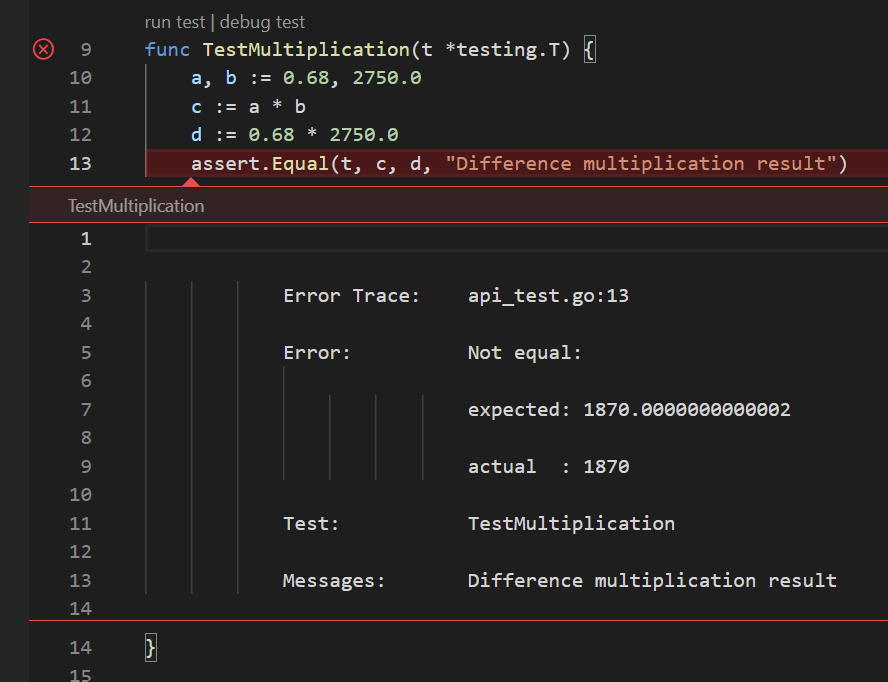### What did you expect to see?

I thought that `c` must be eqal to `d`

### What did you see instead?

In another case i get correct results just like this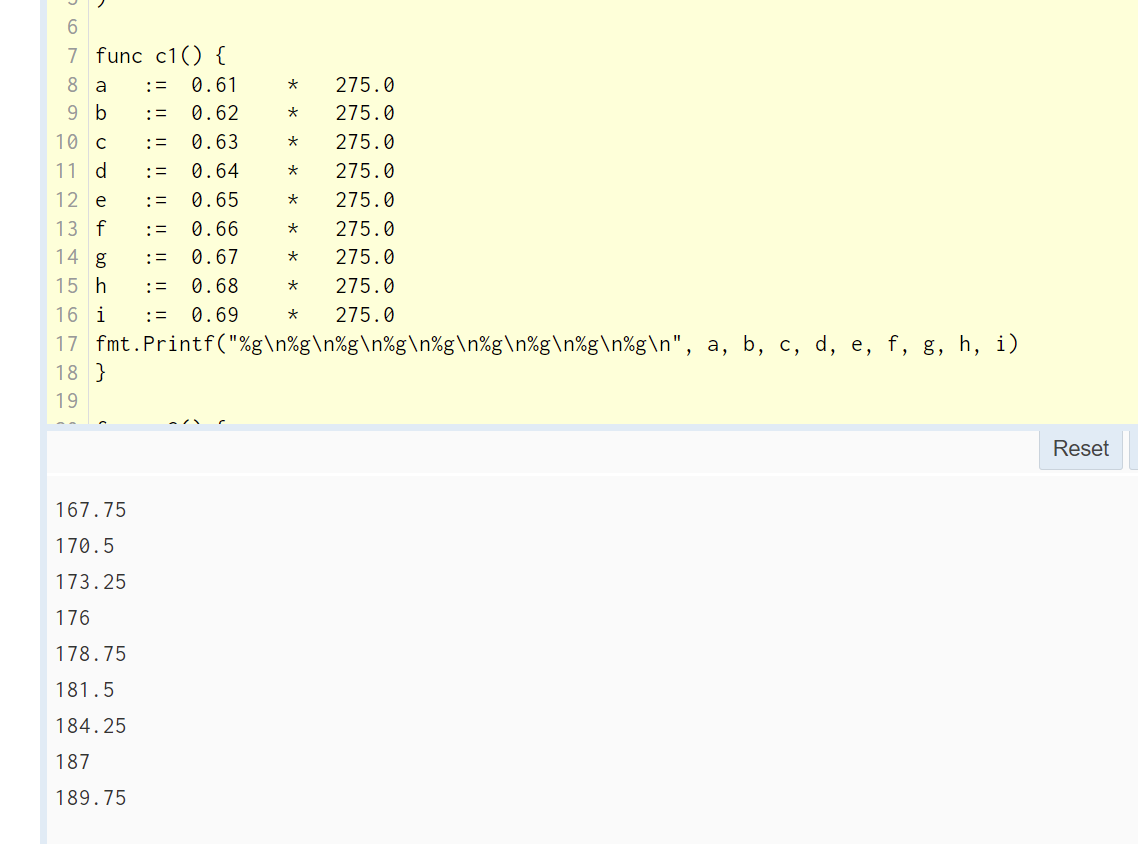but the arithmetic give difference results by this formula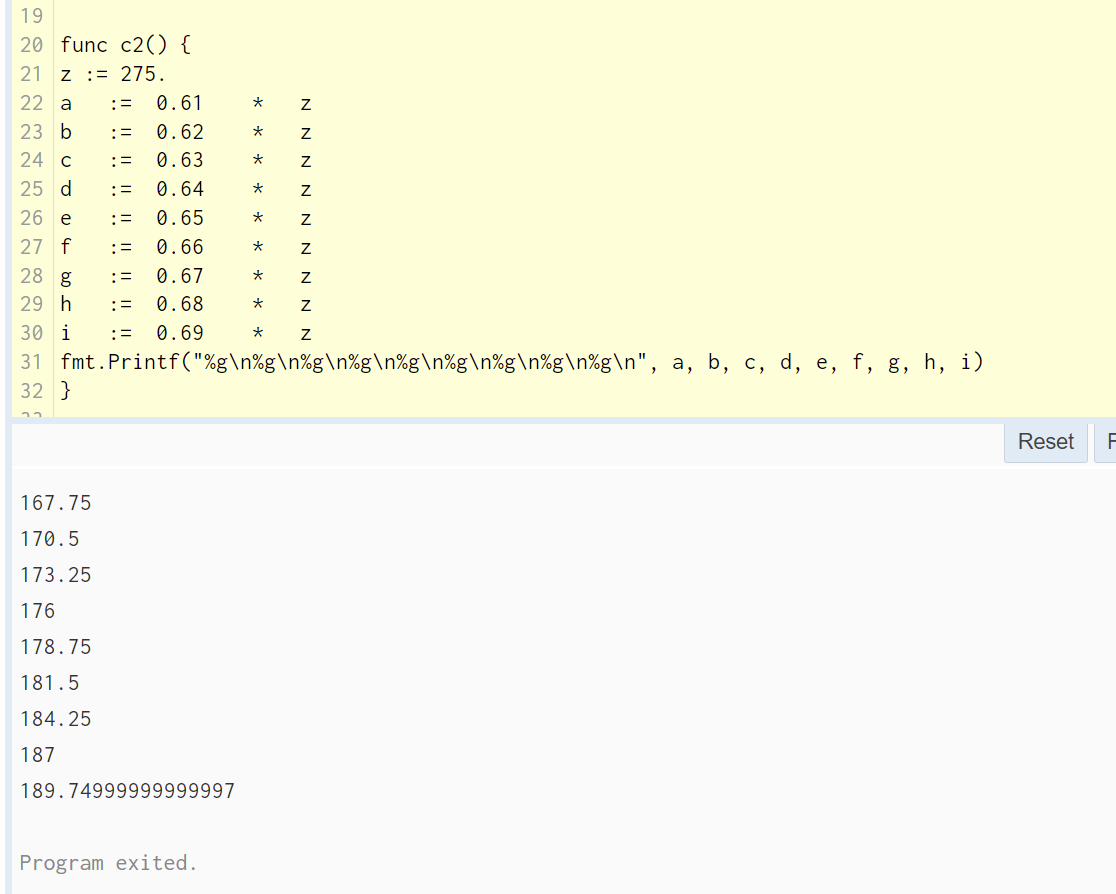I got same issue with division and addition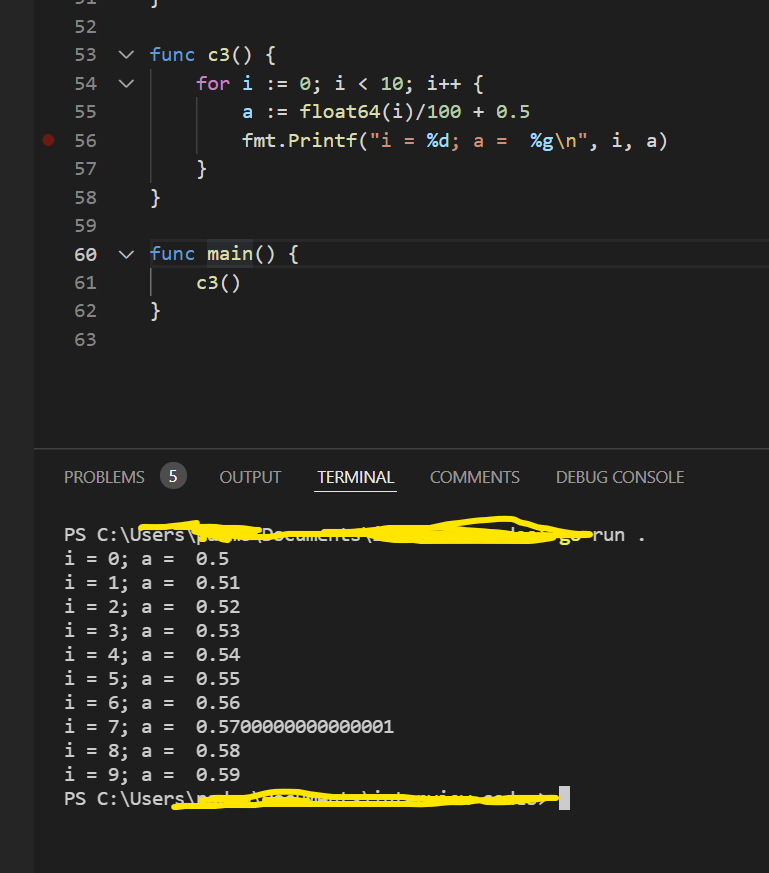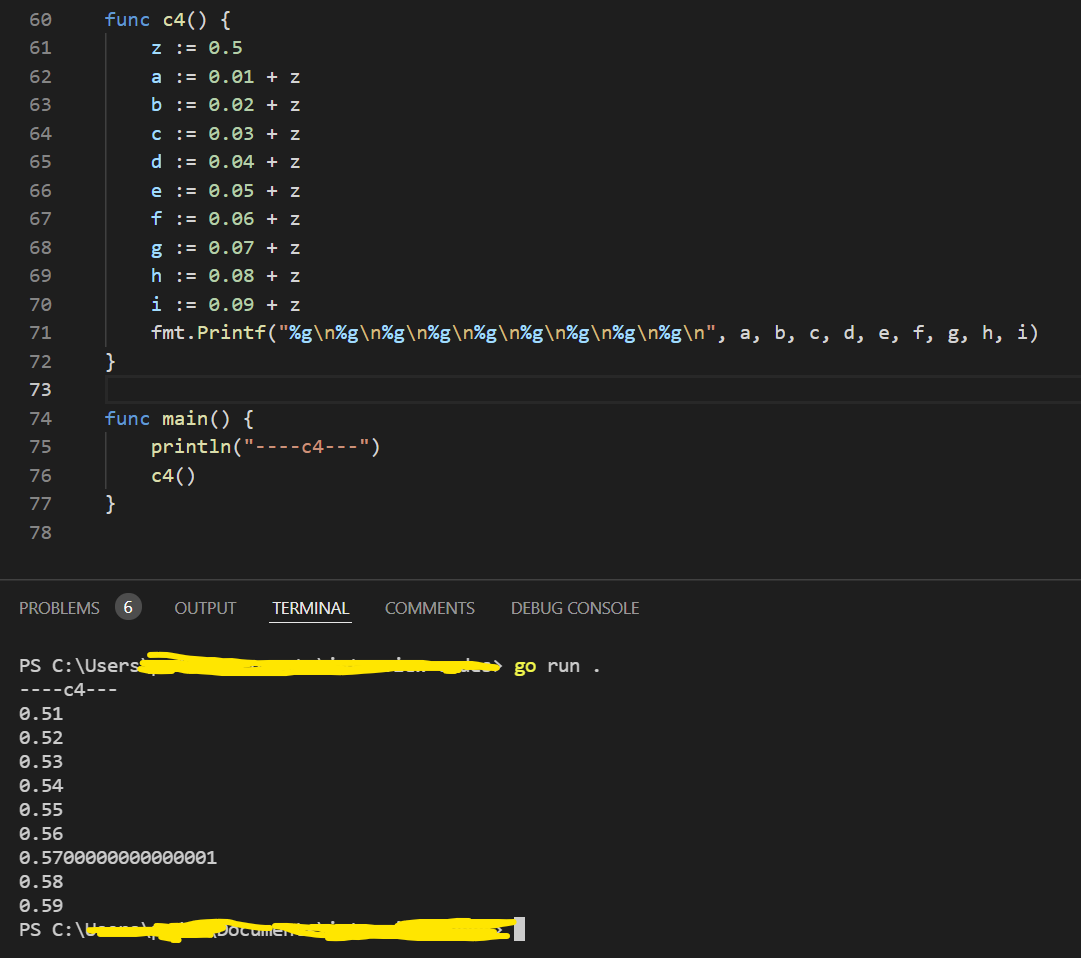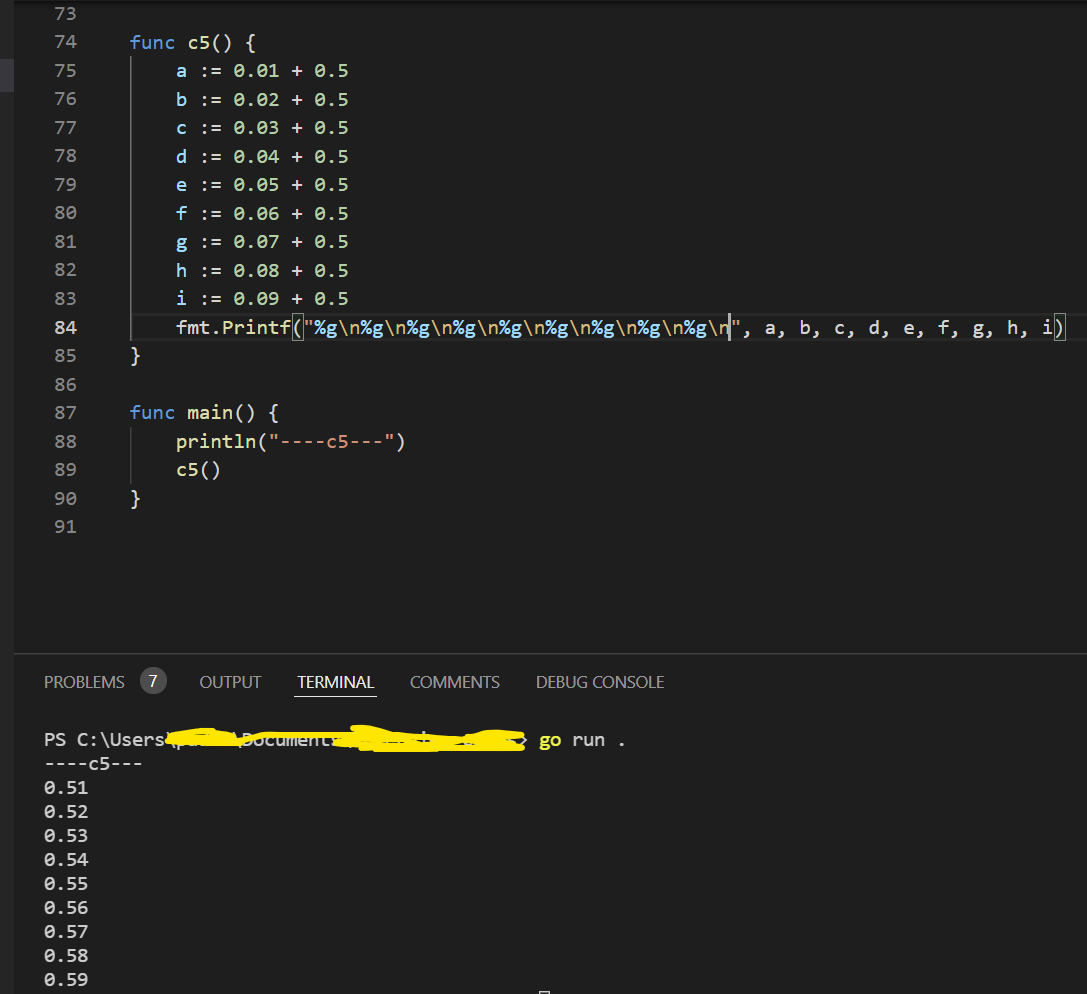``````test codes

func c1() {
a	:=	0.61	*	275.0
b	:=	0.62	*	275.0
c	:=	0.63	*	275.0
d	:=	0.64	*	275.0
e	:=	0.65	*	275.0
f	:=	0.66	*	275.0
g	:=	0.67	*	275.0
h	:=	0.68	*	275.0
i	:=	0.69	*	275.0
fmt.Printf("%g\n%g\n%g\n%g\n%g\n%g\n%g\n%g\n%g\n", a, b, c, d, e, f, g, h, i)
}

func c2() {
z := 275.
a	:=	0.61	*	z
b	:=	0.62	*	z
c	:=	0.63	*	z
d	:=	0.64	*	z
e	:=	0.65	*	z
f	:=	0.66	*	z
g	:=	0.67	*	z
h	:=	0.68	*	z
i	:=	0.69	*	z
fmt.Printf("%g\n%g\n%g\n%g\n%g\n%g\n%g\n%g\n%g\n", a, b, c, d, e, f, g, h, i)
}

func c3() {
for i := 0; i < 10; i++ {
a := float64(i)/100 + 0.5
fmt.Printf("i = %d; a =  %g\n", i, a)
}
}

func c4() {
z := 0.5
a := 0.01 + z
b := 0.02 + z
c := 0.03 + z
d := 0.04 + z
e := 0.05 + z
f := 0.06 + z
g := 0.07 + z
h := 0.08 + z
i := 0.09 + z
fmt.Printf("%g\n%g\n%g\n%g\n%g\n%g\n%g\n%g\n%g\n", a, b, c, d, e, f, g, h, i)
}

func c5() {
a := 0.01 + 0.5
b := 0.02 + 0.5
c := 0.03 + 0.5
d := 0.04 + 0.5
e := 0.05 + 0.5
f := 0.06 + 0.5
g := 0.07 + 0.5
h := 0.08 + 0.5
i := 0.09 + 0.5
fmt.Printf("%g\n%g\n%g\n%g\n%g\n%g\n%g\n%g\n%g\n", a, b, c, d, e, f, g, h, i)
}
``````

###robpike commented Feb 6, 2022

 This is how floating-point calculations work on all computers vs. how constants work in Go. They do not behave the same. Please read these two links:

###ianlancetaylor commented Feb 6, 2022

 Please post plain text as plain text, not as images. Images are difficult to read. Thanks. Closing this issue because it is not a bug in Go. Please comment if you disagree.